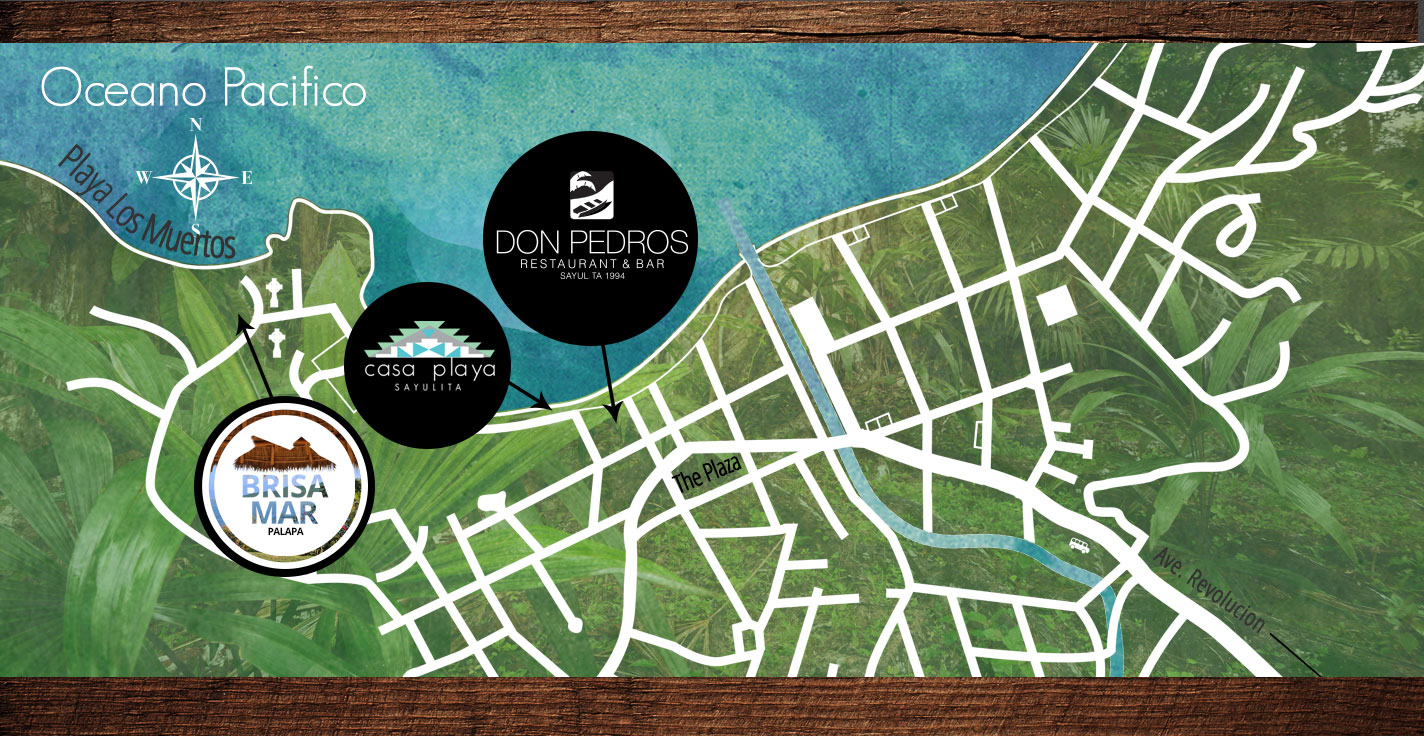1
2
3Don Pedro’s, Calle Marlin 2, Centro, 63734, Sayulita, Nayarit, MEXICO

D O N   P E D R O ‘ S    R E S T A U R A N T   M A I N   F L O O R

D O N   P E D R O ‘ S    R E S T A U R A N T   S E C O N D   L E V E L

B R I S A    M A R    P A L A P A

C A S A    P L A Y A